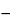Aluminum Dichlorohydrate
(a loo' mi num dye klor'' oh hye' drate).
Aly(OH)3y-zClz·nH2O

Aluminum chlorohydroxide.
Aluminum hydroxychloride.
» Aluminum Dichlorohydrate consists of complex basic aluminum chloride that is polymeric and loosely hydrated and encompasses a range of aluminum-to-chloride atomic ratios between 0.90:1 and 1.25:1. It contains not less than 90.0 percent and not more than 110.0 percent of the labeled amount of anhydrous aluminum dichlorohydrate.
Packaging and storage— Preserve in well-closed containers.
Labeling— The label states the content of anhydrous aluminum dichlorohydrate.
Identification—
between 3.0 and 5.0, in a solution [15 in 100 (w/w)].
Limit of iron— Using Aluminum Dichlorohydrate instead of Aluminum Chlorohydrate, proceed as directed in the test for Limit of iron under Aluminum Chlorohydrate. The limit is 150 µg per g.
Content of aluminum— Using Aluminum Dichlorohydrate instead of Aluminum Chlorohydrate, proceed as directed in the test for Content of aluminum under Aluminum Chlorohydrate. Use the result obtained to calculate the Aluminum/chloride atomic ratio.
Content of chloride— Using Aluminum Dichlorohydrate instead of Aluminum Chlorohydrate, proceed as directed in the test for Content of chloride under Aluminum Chlorohydrate. Use the result obtained to calculate the Aluminum/chloride atomic ratio.
Aluminum/chloride atomic ratio— Divide the percentage of aluminum found in the test for Content of aluminum by the percentage of chloride found in the test for Content of chloride and multiply by 35.453/26.98, in which 35.453 and 26.98 are the atomic weights of chlorine and aluminum, respectively: the ratio is between 0.90:1 and 1.25:1.
Assay— Calculate the percentage of anhydrous aluminum dichlorohydrate in the Aluminum Dichlorohydrate by the formula:
Al({26.98x + [17.01(3x1)] + 35.453} / 26.98x)
in which Al is the percentage of aluminum found in the test for Content of aluminum, x is the aluminum/chloride atomic ratio, 26.98 is the atomic weight of aluminum, 17.01 is the molecular weight of the hydroxide anion (OH), and 35.453 is the atomic weight of chlorine (Cl).
Auxiliary Information— Please check for your question in the FAQs before contacting USP.
Topic/Question Contact Expert Committee
Monograph Feiwen Mao, M.S.
Senior Scientific Liaison
1-301-816-8320
(SM32010) Monographs - Small Molecules 3
USP35–NF30 Page 2129